## Introduction

Protons jump among molecules in water and ice. The proton dynamics in water and aqueous solutions has been a major subject of research in physical chemistry for the life sciences. Similarly, the proton dynamics in ice has been a major subject of research in the earth and planetary sciences. Grotthuss proposed the proton relay model for water in 18061 and Bjerrum proposed the rotational defect model for ice in 19522. There have been innumerable experiments concerning the proton conduction in ice for more than a century3 and the corresponding theory has been established through the efforts of multiple researchers4,5,6. In most cases, temperature effects and impurity effects have been addressed, whereas pressure effects7,8,9,10,11 have been addressed in only a few cases. Water has a complex phase diagram with many phases (Fig. 1). Under compression at room temperature, liquid water freezes into ice VI at approximately 1 GPa and transforms into ice VII at pressures over 2 GPa12,13. Ice VII is a crystal of water molecules formed into a bcc oxygen lattice and a random hydrogen-bond network, which is composed of two interpenetrating but independent ice Ic sublattices. As the pressure increases from 2 GPa to 60 GPa, the bcc oxygen lattice remains stable with a reduced lattice constant, while the hydrogen-bond network evolves from a static random state to a dynamic random state and eventually to a symmetric state. Ice VII exhibits many phenomena originating from its complex proton dynamics, regarding which X-ray diffraction provides very little information. Among these phenomena, superionic ice14 is a theoretical phase of water under high temperature and high pressure that is composed of ionised water and has the properties of both a crystal and a liquid: the oxygen ions crystallise in the bcc (or fcc15, at extremely high pressures) lattice, whereas the hydrogen ions flow inside the oxygen lattice. It has been suggested that ice-giant planets, such as Uranus and Neptune, may possess a layer of superionic ice16. However, experimental evidence of superionic ice has been elusive, partly because of the lack of a clear-cut definition of the phase. Cavazzoni et al.14 have identified superionic ice in quantum molecular dynamics simulations by observing an abrupt increase in the proton-diffusion coefficient. Sugimura et al.17 have defined it as a state of ice VII with electrical conductivity greater than 10−1 (S/m) because the observed conductivity was found to follow a single Arrhenius-type relation and did not exhibit any discontinuity. Goncharov et al.16 have suggested a new phase observed at greater than 47 GPa via Raman spectroscopy to be superionic ice, although no information regarding the proton dynamics was available. Therefore, no phase boundary for superionic ice has yet been confirmed experimentally. Originally, the present study was initiated to investigate the existence of superionic ice by probing proton conduction using the impedance spectroscopy method; however, as reported in this article, an unexpected peak was discovered in the proton conductivity of ice VII measured in the pressure range between 2 GPa and 40 GPa at room temperature. The implications of this discovery are discussed here.

## Results

In the present experiment, high-pressure conditions were generated using a diamond anvil cell (DAC) with flat faces of 0.35 mm in diameter. Distilled and deionised H2O water was loaded into a hole drilled into an electrically insulating gasket composed of cubic boron nitride (cBN) and rhenium (Re)18. Two electrodes made of platinum (Pt) foil with a thickness of 0.002 mm were placed on the cBN layer (Fig. 2a) in runs #1–5, whereas gold (Au) electrodes were sputtered onto the culet surfaces of both diamonds (Fig. 2b) in runs #6–9. The structure of the apparatus is depicted in Supplemental Fig. S1. The width and length of the Pt leads attached to the cBN layer indicated in the figure were approximately 0.2 mm and 1.5 mm, respectively. The thickness of the cBN layer was not measured but was estimated to be 0.05 mm. The pressure was measured by observing the Raman shift of the high-frequency edge of the stressed diamonds19. No electrical contact between the two electrodes or between the electrodes and the gasket was detected at any stage in any experiment. The complex impedance of the system — Z(ω) = Z′(ω) + iZ″(ω), where Z′(ω) and Z″(ω) are the real and imaginary parts, respectively — was measured at room temperature and at pressures from 2.2 GPa to 40 GPa; for these measurements, the quasi-four-terminal method was employed at an applied voltage of 1 V and frequencies, f, from 20 Hz to 1 MHz using an Agilent 4284A precision LCR meter. The obtained impedance data is presented as a Cole-Cole plot (Fig. 3) and was fitted using an equivalent circuit, whose impedance, Z, consisted of a sample contribution (R1,ZCPE), a parasitic contribution (R2,C2) and an electrode contribution (R0):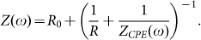A constant phase element20,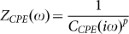, is an equivalent electrical circuit component that models an imperfect capacitor with fitting parameters and it reduces to an ideal capacitor C when p = 1 and CCPE= C. The equivalent capacitance for a CPE is equal to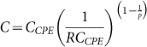.

Data collected at frequencies lower than ~100 Hz are strongly influenced by the surface effect of the electrodes, as shown in Fig. 3. Note that the diameter of the semi-circle in the Cole-Cole plot indicates the resistance, R, independent of ZCPE(ω) and C2.

Supplementary Fig. S2 presents the pressure dependence of the resistance, R and the capacitance, C. There is significant scatter in the data from run to run because of the change in the electrode configuration and, most likely, surface effects. Nevertheless, a common trend can be seen: the resistance exhibits a minimum at Pc and the capacitance exhibits both a maximum and a minimum near Pc.

This trend can be seen more clearly in Supplementary Fig. S3, where the effect of the sample-size variation is reduced by plotting the conductivity, σ = g/R and permittivity, ε = gC, using the geometric factor g = (d/A) for an ideal parallel-plate capacitor with a cross section A and a distance d. Because this geometric factor is exact only for Ad2, a more accurate geometric factor was evaluated using the boundary element method (BEM)21 with the electrodes modelled as rectangular plates immersed in uniform dielectrics. The pressure dependence of the result is consistent with the theoretical prediction10,11: the conductivity exhibits a clear peak at Pc ≈ 10 GPa (Fig. 4a) and the permittivity, ε, exhibits a minimum near Pc (Fig. 4b). The magnitude of the permittivity, however, seems to be overestimated probably because of the contribution from the parasitic capacitance and the difficulty in evaluating the accurate geometric factor taking the apparatus structure into account. The activation energy for the conductivity was evaluated based on the conductivities measured during the processes of cooling and heating between 278 K and 303 K at several constant loads. The activation energy reaches a minimum of 0.49 eV near 10 GPa (Fig. 4c).

It is worth noting that the trend in pressure dependence is already recognisable in the resistance and capacitance, as the change in geometry is much smoother than the exponential change in conductivity and permittivity.

Supplementary Fig. S4 presents the cross section, A and the distance, d, measured at each pressure and in each run. In runs #1–5, in which the two platinum electrodes were arranged in skew positions (Fig. 2a), d and A were measured using an optical microscope. In runs #6–9, in which the two orthogonal gold electrodes were sputtered onto the culet surfaces (Fig. 2b), the cross section, A, is defined as the overlapping area of the two electrodes as seen from the pressurising axial direction and was measured at each pressure using an optical microscope. The distance, d, is defined as the interval between the culets of the diamond anvils, neglecting the thickness of the sputtered gold electrodes and was measured using the white-light interference method and the published reflective index of high-pressure ice22.

Because the contributions of the sample and those of parasitic effects are indistinguishable in a single run, the impedance of an empty cell with gold electrodes was measured in a separate run (Supplemental Fig. S5) and the parasitic contributions were found to remain nearly constant during the run at R2 = 9 × 109 (Ω) and C2 = 2 × 10−13 (F). These values are in good agreement with the theoretical estimates,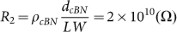and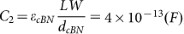, which were modelled for a parallel-plate capacitor consisting of a cBN layer (with a thickness of dcBN = 5 × 10−5(m), a resistivity23 of ρcBN = 108m) and a permittivity23 of εcBN0 = 7.1) sandwiched between a Re gasket and Pt leads (with a length of L = 1.5 × 10−3(m) and a width of W = 2 × 10−4(m)), as shown in Supplemental Fig. S1. This result implies that the majority of the parasitic contributions originate from this circuit. This knowledge will be useful for the optimisation of the designs of future experiments. In the pressure range between 2 GPa and 20 GPa, where RR1 R2, the measured resistance R is approximately equal to the sample contribution, whereas above 20 GPa, it is nearly equal to the parasitic contributions. The parasitic contribution of the capacitance is nearly on the order of the sample contribution; which one is larger than the other depends on the experimental conditions of the given run. If CC1 C2, then the sample contribution is measured and a maximum and minimum in the capacitance appear near Pc. If CC2 C1, then the parasitic contribution is measured and the capacitance exhibits a monotonic pressure dependence. A similar argument can also be applied to the cells with platinum electrodes (Fig. 2a), although their empty-cell impedance was not measured in this study.

## Discussion

According to theoretical studies10,11, this peak in the conductivity can be attributed to the transition of the major charge carriers in ice VII from the rotational defects to the ionic defects. The theory of electrical conduction in ice5 describes such electrical conduction in terms of excitations of proton-defect pairs from the ground state of the hydrogen-bond network4,5 that satisfy the ice rules24. The conduction mechanism is analogous to that of electrical conduction in an intrinsic semiconductor, which can be described in terms of excitations of electron-hole pairs. The unique feature of electrical conduction in ice is that two types of defect pairs are involved4,5, i.e., ionic defect pairs (H3O+ and OH) and rotational defect pairs (L-defects and D-defects)2. The former breaks the first ice rule, “two hydrogen atoms exist adjacent to each oxygen atom”. The latter breaks the second rule, “exactly one hydrogen atom exists in a bond”. Because proton conduction requires both types of defect pairs, the electrical conductivity (σ) is determined by the smaller of the ionic (σ±) and rotational (σDL) defect conductivities3,4.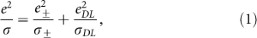where e and eα are the charges of the proton and the defect α, respectively. If it is assumed that the density and diffusion constant of the defects follow an Arrhenius-type relation, the defect conductivity can be expressed as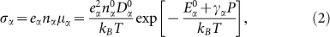where nα and μα are the number density and mobility of defect α, respectively. The defect charges are e± = 0.62e and eDL = 0.38e for ice Ih3. The constants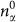and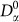are the prefactors of the density and the diffusion constant, respectively;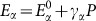is the activation energy for the conductance attributable to defect α at a pressure P, where γα is the activation volume. In general, γ± < 0 because the barrier between the two possible hydrogen positions in a bond decreases as the pressure increases, whereas γDL > 0 because a hydrogen bond becomes stronger as the pressure increases. These parameters have been estimated11 to be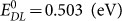,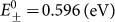, γDL = 2.59 × 10−2 (eV/GPa), γ± = −3.64 × 10−2 (eV/GPa),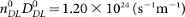and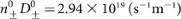by fitting experimental results9,25 obtained at low pressure. The permittivity ε = ε + ε0χS of ice can be represented in terms of the electrical susceptibility,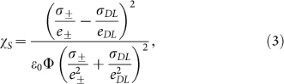where Φ is a constant3. A remarkable feature of eq. (3) is that χS becomes zero when σ±/e± = σ±/eDL because the fluxes of the two types of defects are balanced, allowing electrical current to flow without any build-up of polarisation3. The features observed in the measured data can be explained using this model. According to eq. (1), the conductivity reaches a maximum at a certain critical pressure, Pc ≈ 10 GPa, where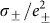and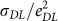become equal. The permittivity has both a maximum and a minimum near Pc, as seen from eq. (3). The activation energy for σ is approximately equal to E± below Pc and is approximately equal to EDL above Pc, which explains the minimum at Pc.

Molecular dynamics (MD) simulations11 can provide deeper insight into the microscopic mechanism of this conductivity peak. In the MD simulations, the conductivity was evaluated based on the mean-square deviation (MSD) of the protons: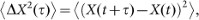where X(t) is the position of a proton at time t and a pair of brackets indicates the average over all trajectories. A linear increase in MSD with time indicates diffusion motion with the diffusion constant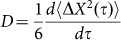. The calculated MSDs are presented in Supplementary Fig. S6. Each MSD consists of two linear components, implying that two diffusion processes are involved: Below Pc, protons rotate with the water molecules around the oxygen lattice sites and occasionally jump to neighbouring molecules; this behaviour corresponds to the rapid linear increase in the MSD to the size of a water molecule, followed by a slower linear increase. Above Pc, protons oscillate in hydrogen bonds and occasionally jump to neighbouring bonds; this behaviour corresponds to the rapid linear increase to the distance between the two stable positions of protons in hydrogen bonds, followed by a slower linear increase. The electrical conductivity was evaluated as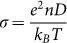, where n is the density of protons and a pressure dependence similar to that observed experimentally and through thermodynamic modelling was obtained. In all simulations, the MSD of the oxygen atoms remained on the order of atomic thermal vibrations, indicating that the oxygen atoms remain at the lattice sites.

The effects of pressure on the conductivity of ice Ih have been studied by a number of researchers7,8,9. Hubmann8 has measured the conductivity of doped ice Ih from ambient pressure to the phase boundary between ice Ih and liquid water (0.15 GPa). The total conductivity could be decomposed into ionic and rotational components, with γ± < 0 and γDL > 0, respectively. The transition of the charge carriers was not observed at −25°C because the theoretical peak pressure was above the range of stability for ice Ih. The conductivity peak became observable at −51°C and −61°C because the peak pressure decreases as the temperature decreases, thus moving into the stability region for ice Ih. By contrast, the conductivity peak of pure ice VII can be observed even at room temperature by virtue of its much wider pressure range of stability.

The observation of a conductivity peak attributable to a pressure-induced carrier transition in ice VII is reported here for the first time. Previous measurements9,17,26 of electrical conductivity (or the diffusion constant) are consistent with the present result; however, these previous measurements were limited to pressure ranges either below or above Pc. Zheng et al.9 have measured the conductivity of ice and water between 0.21 GPa and 4.18 GPa and found that the conductivity decreases from 10−5 S/m at 1 GPa to 10−6 S/m at 2 GPa in ice VI and then begins to increase at 2 GPa in ice VII. The proton-diffusion constant in ice VII at 400 K has been measured using infrared reflection spectroscopy by Katoh et al.26. The diffusion constant was found to decrease exponentially from 10−15 (m2/s) at 10 GPa to 10−17 (m2/s) at 63 GPa. Sugimura et al.17 have measured the conductivity of ice VII at temperatures up to 873 K and pressures between 20 GPa and 101 GPa.

In summary, we discovered an unexpected peak in the electrical conductivity of ice VII as measured through impedance spectroscopy in a diamond anvil cell (DAC) during the process of compression from 2 GPa to 40 GPa at room temperature. This peak can be attributed to the transition of the major charge carriers in ice VII from the rotational defects to the ionic defects.

## Methods

### Molecular dynamics simulations

In the molecular dynamics simulations, an empirical water-potential model with all degrees of freedom27,28 was employed, instead of the widely used rigid-molecule models29, to account for the ionisation of water molecules. The simulation cell for ice VII consisted of 128 water molecules and the atom trajectories were typically calculated for up to 500 ps at pressures between 2 GPa and 60 GPa and at a temperature of 800 K. The temperature was set higher to account for the quantum zero-point motion of the protons, which effectively lowers the diffusion barrier. Therefore, the simulations were not fully quantum and the results should be taken to be semi-quantitative rather than numerically rigorous. The particle trajectories were computed using the Verlet algorithm30 and temperature was controlled by a Nose thermostat31. The lattice constant of the cubic simulation cell was fixed to the value calculated via static geometric optimisation within the DFT theory32 at a given pressure P.